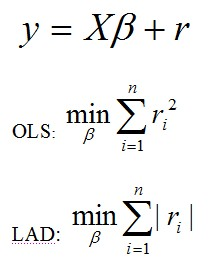# LAD: An Alternative to OLS

Statistical Analysis Techniques
, Robust Estimators, Alternatives to OLS

Least absolute deviation (LAD) (also known as least absolute residuals, least absolute errors and least absolute value) is an alternative method to the conventional ordinary least squares (OLS), for building regression models. Instead of estimating the coefficients that minimise the sum of squared residuals, LAD estimates the coefficients that minimises the sum of the absolute residuals.Compared to OLS, LAD has the advantage of being resistant to outliers and robust to departures from the normality assumption.

The disadvantages of LAD include being more computationally expensive than OLS and having the possibility of more than one solution.  The reason for this is that unlike OLS (which has an analytical solution derived from matrix calculus), the LAD coefficient estimates are found iteratively, usually with the OLS estimates as the initial values.

LAD belongs to a broader class of robust estimators known as M-estimators.